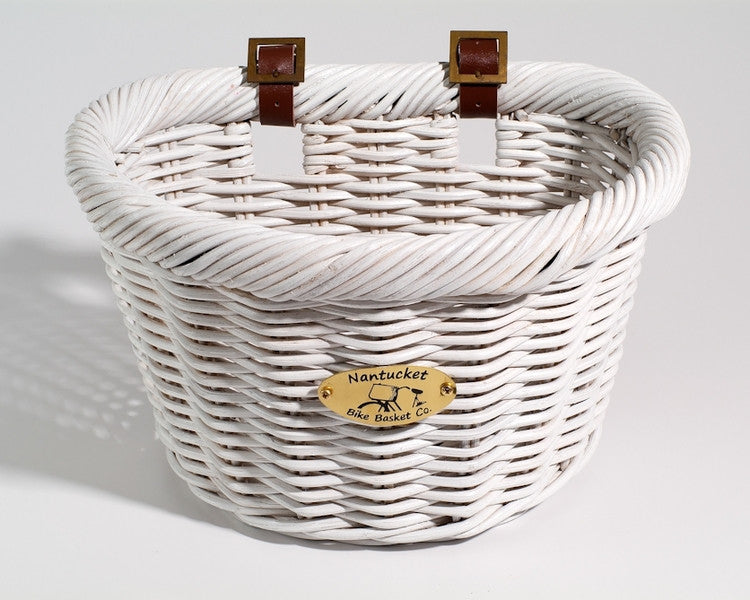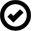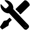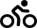Liquid error: Could not find asset snippets/spurit_po-product-snippet.liquid# Nantucket Cruiser Collection Front Wicker Baskets

#### Video

Our Cruiser baskets are made from the highest quality natural rattan fibers. Woven from pitrit rattan, the extra thick weave of this collection holds up well to the demands of daily use. Attaches to front handlebars with two leather straps.

Dimensions: 14.5" (L) x 10.5" (W) x 9.5" (H), Maximum Weight Limit: 13 pounds

Dimensions: 14.5" (L) x 10.5" (W) x 9.5" (H), Maximum Weight Limit: 13 pounds

Dimensions: 14.5" (L) x 10.5" (W) x 9.5" (H), Maximum Weight Limit: 13 pounds

Dimensions: 14.5" (L) x 10.5" (W) x 9.5" (H), Maximum Weight Limit: 13 pounds

Dimensions: 14.5" (L) x 10.5" (W) x 9.5" (H), Maximum Weight Limit: 13 pounds

Dimensions: 14.5" (L) x 10.5" (W) x 9.5" (H), Maximum Weight Limit: 13 pounds

Dimensions: 14.5" (L) x 10.5" (W) x 9.5" (H), Maximum Weight Limit: 13 pounds

Dimensions: 14.5" (L) x 10.5" (W) x 9.5" (H), Maximum Weight Limit: 13 pounds

Dimensions: 14.5" (L) x 10.5" (W) x 9.5" (H), Maximum Weight Limit: 13 pounds

Dimensions: 12.5" (L) x 8.25" (W) x 8.5" (H), Maximum Weight Limit: 13 pounds

Our Cruiser baskets are made from the highest quality natural rattan fibers. Woven from pitrit rattan, the extra thick weave of this collection holds up well to the demands of daily use. Attaches to front handlebars with two leather straps.

Dimensions: 14.5" (L) x 10.5" (W) x 9.5" (H), Maximum Weight Limit: 13 pounds

Dimensions: 14.5" (L) x 10.5" (W) x 9.5" (H), Maximum Weight Limit: 13 pounds

Dimensions: 14.5" (L) x 10.5" (W) x 9.5" (H), Maximum Weight Limit: 13 pounds

Dimensions: 14.5" (L) x 10.5" (W) x 9.5" (H), Maximum Weight Limit: 13 pounds

Dimensions: 14.5" (L) x 10.5" (W) x 9.5" (H), Maximum Weight Limit: 13 pounds

Dimensions: 14.5" (L) x 10.5" (W) x 9.5" (H), Maximum Weight Limit: 13 pounds

Dimensions: 14.5" (L) x 10.5" (W) x 9.5" (H), Maximum Weight Limit: 13 pounds

Dimensions: 14.5" (L) x 10.5" (W) x 9.5" (H), Maximum Weight Limit: 13 pounds

Dimensions: 14.5" (L) x 10.5" (W) x 9.5" (H), Maximum Weight Limit: 13 pounds

Dimensions: 12.5" (L) x 8.25" (W) x 8.5" (H), Maximum Weight Limit: 13 pounds

Our Cruiser baskets are made from the highest quality natural rattan fibers. Woven from pitrit rattan, the extra thick weave of this collection holds up well to the demands of daily use. Attaches to front handlebars with two leather straps.

Dimensions: 14.5" (L) x 10.5" (W) x 9.5" (H), Maximum Weight Limit: 13 pounds

Dimensions: 14.5" (L) x 10.5" (W) x 9.5" (H), Maximum Weight Limit: 13 pounds

Dimensions: 14.5" (L) x 10.5" (W) x 9.5" (H), Maximum Weight Limit: 13 pounds

Dimensions: 14.5" (L) x 10.5" (W) x 9.5" (H), Maximum Weight Limit: 13 pounds

Dimensions: 14.5" (L) x 10.5" (W) x 9.5" (H), Maximum Weight Limit: 13 pounds

Dimensions: 14.5" (L) x 10.5" (W) x 9.5" (H), Maximum Weight Limit: 13 pounds

Dimensions: 14.5" (L) x 10.5" (W) x 9.5" (H), Maximum Weight Limit: 13 pounds

Dimensions: 14.5" (L) x 10.5" (W) x 9.5" (H), Maximum Weight Limit: 13 pounds

Dimensions: 14.5" (L) x 10.5" (W) x 9.5" (H), Maximum Weight Limit: 13 pounds

Dimensions: 12.5" (L) x 8.25" (W) x 8.5" (H), Maximum Weight Limit: 13 pounds•••